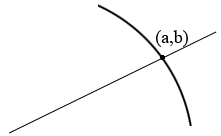SEARCH HOMEMath Central Quandaries & QueriesQuestion from Roxanne: Hello, i need to ask a question, do you mind explaining and writing the formula of how to solve equations such as "find the set values of k for which the line y=2x-5 cuts the curve y=x^2 +kx+11" pleaseHi Roxanne,

I always draw pictures. Here is my picture of a line and a curve and a line where the line cuts the curve. I let $(a,b)$ be the point where the line cuts the curve.Since $(a,b)$ lies on the line it satisfies the equation of the line and hence

$b = 2a - 5.$

The point $(a,b)$ is also on the curve so

$b = a^2 + ka + 11.$

Hence

$2a - 5 = a^2 + ka + 11.$

For what range of values of $k$ does the quadratic above have real roots?

Penny* Registered trade mark of Imperial Oil Limited. Used under license.Math Central is supported by the University of Regina and the Imperial Oil Foundation.